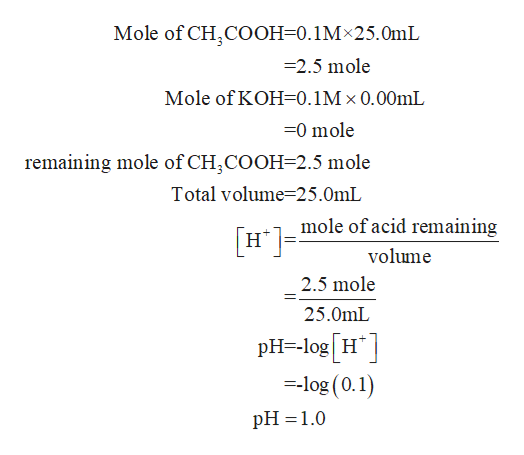Question
21 views
check_circle

Step 1

Given that,

Mass of acetic acid = 0.1 M

Volume of acetic acid= 25mL

Mass of KOH = 0.1 M

Step 2

A: when 0.00 mL of KOH is added

Firstly we calculate the molehelp_outlineImage TranscriptioncloseMole of CH,COOH-0.1M 25.0mL 2.5 mole Mole of KOH 0.1M x 0.00mL -0 mole remaining mole of CH,COOH-2.5 mole Total volume 25.0mL mole of acid remaining Н" volume 2.5 mole 25.0mL pH-log [H -log (0.1) н" pH 1.0 fullscreen
Step 3

B: When 12.5mL of KOH added

Mole of acetic acid = 2.5 mole

Mole of KOH = 1.25 mol

1.25 moles of both w...

### Want to see the full answer?

See Solution

#### Want to see this answer and more?

Solutions are written by subject experts who are available 24/7. Questions are typically answered within 1 hour.*

See Solution
*Response times may vary by subject and question.
Tagged in

### Chemistry